Chemistry - 2015 Past Year Paper

# Chemistry - 2015 Past Year Paper - IIT JAM

Test Description

## 60 Questions MCQ Test IIT JAM Past Year Papers and Model Test Paper (All Branches) - Chemistry - 2015 Past Year Paper

Chemistry - 2015 Past Year Paper for IIT JAM 2023 is part of IIT JAM Past Year Papers and Model Test Paper (All Branches) preparation. The Chemistry - 2015 Past Year Paper questions and answers have been prepared according to the IIT JAM exam syllabus.The Chemistry - 2015 Past Year Paper MCQs are made for IIT JAM 2023 Exam. Find important definitions, questions, notes, meanings, examples, exercises, MCQs and online tests for Chemistry - 2015 Past Year Paper below.
Solutions of Chemistry - 2015 Past Year Paper questions in English are available as part of our IIT JAM Past Year Papers and Model Test Paper (All Branches) for IIT JAM & Chemistry - 2015 Past Year Paper solutions in Hindi for IIT JAM Past Year Papers and Model Test Paper (All Branches) course. Download more important topics, notes, lectures and mock test series for IIT JAM Exam by signing up for free. Attempt Chemistry - 2015 Past Year Paper | 60 questions in 180 minutes | Mock test for IIT JAM preparation | Free important questions MCQ to study IIT JAM Past Year Papers and Model Test Paper (All Branches) for IIT JAM Exam | Download free PDF with solutions
 1 Crore+ students have signed up on EduRev. Have you?
Chemistry - 2015 Past Year Paper - Question 1

### The first row transition metal complexes having tetrahedral geometry are high- spin due to

Detailed Solution for Chemistry - 2015 Past Year Paper - Question 1

Tetrahedral complexes always show high spin complex i.e. no pairing of electrons because in tetrahedral complexes the coordination number of metal is four only, thus crystal field splitting is less in tetrahedral complexes. Hence, pairing energy is more compared to the crystal field splitting energy.

Chemistry - 2015 Past Year Paper - Question 2

### The species responsible for the superacidity of SbF5- HSO3F system is

Chemistry - 2015 Past Year Paper - Question 3

### A filter paper moistened with cadmium acetate solution turns yellow upon exposure to H2S. The transition responsible for the yellow colour is

Chemistry - 2015 Past Year Paper - Question 4

Low- spin iron(III) center is present in

Chemistry - 2015 Past Year Paper - Question 5

The major product formed in the following reaction is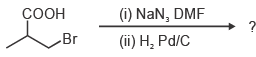Chemistry - 2015 Past Year Paper - Question 6

The structure of (2S,3R)- 2- amino- 3- hydroxy butanoic acid is

Chemistry - 2015 Past Year Paper - Question 7

The ene- yne that produces a chiral compound upon treatment with Lindlar’s catalyst is

Chemistry - 2015 Past Year Paper - Question 8

An organic compound P (C4H8O) is positive to Bayer¡¦s test, but inert to sodium metal. On treatment with conc. HCl, P gives CH3CH2Cl and CH3CHO. The structure of P is

Chemistry - 2015 Past Year Paper - Question 9

Which one of the following is an identity matrix?

Chemistry - 2015 Past Year Paper - Question 10

The intermolecular van der Waals potential is inversely proportional to r6. The corresponding force is proportional to

Chemistry - 2015 Past Year Paper - Question 11

The normal spinel among the following mixed metal- oxides is

Chemistry - 2015 Past Year Paper - Question 12

The ground state term for a free ion with 3d7 configuration is

Chemistry - 2015 Past Year Paper - Question 13

The reagent ‘oxine’ commonly used in analytical chemistry is

Chemistry - 2015 Past Year Paper - Question 14

The species having trigonal pyramidal shape is

Chemistry - 2015 Past Year Paper - Question 15

The correct statement about ionization potential (IP) is

Chemistry - 2015 Past Year Paper - Question 16

Mass of one molecule of sulphuric acid is

Chemistry - 2015 Past Year Paper - Question 17

The set of products formed in the following reaction is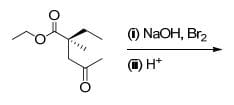Chemistry - 2015 Past Year Paper - Question 18

The correct set of reagents required for the following transformation is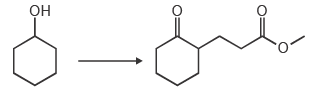Chemistry - 2015 Past Year Paper - Question 19

The correct order of the pKa values for the conjugate acids of heterocyclic compounds given below is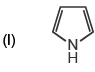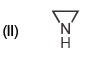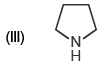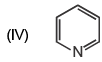Chemistry - 2015 Past Year Paper - Question 20

The correct order of the 1H NMR chemical shift values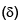for the indicated hydrogens (in bold) in the following compounds is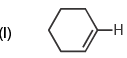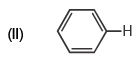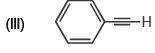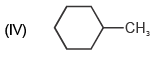Chemistry - 2015 Past Year Paper - Question 21

Which of the following statements are correct for SNAr reaction?

(i) Follows second order kinetics
(ii) KH/KD > 1
(iii) Involves carbanion- type intermediate
(iv) Involves two transition states

Chemistry - 2015 Past Year Paper - Question 22

According to the equipartition principle, the predicted high temperature limiting value of the molar heat capacity at constant volume for C2H2 is

Chemistry - 2015 Past Year Paper - Question 23

The major product formed in the following reaction is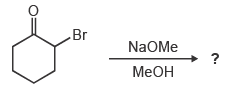Chemistry - 2015 Past Year Paper - Question 24

At 25 °C, the solubility product (Ksp) of CaF2 in water is 3.2 × 10–11. The solubility (in mole per kg of water) of the salt at the same temperature (ignore ion pairing) is

Chemistry - 2015 Past Year Paper - Question 25

For an isothermal free expansion of an ideal gas into vacuum, which one of the following set of values is correct?

Chemistry - 2015 Past Year Paper - Question 26

The kinetics of the reaction 2N2O5 → 4NO2 + O2 in liquid bromine medium was measured independently for three different initial concentrations of N2O5: 0.11, 0.07 and 0.05 mol L–1. The half- life of the reaction was found to be 4.5 hours for all these concentrations. The order of the reaction is

Chemistry - 2015 Past Year Paper - Question 27

The concentration of K+ ion inside a biological cell is 20 times higher than outside.The magnitude of potential difference between the two sides is [Given: 2.303 RT/F = 59 mV]

Chemistry - 2015 Past Year Paper - Question 28

The correct order of the fundamental vibrational frequencies of the following diatomic molecules is

Chemistry - 2015 Past Year Paper - Question 29

Identify the correct reagents required for the following transformation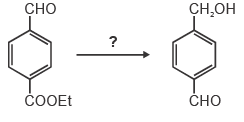Chemistry - 2015 Past Year Paper - Question 30

The complex that is expected to show orbital contribution to the overall magnetic moment is

*Multiple options can be correct
Chemistry - 2015 Past Year Paper - Question 31

The common feature(s) of Rb+, Kr and Br is/are that they

*Multiple options can be correct
Chemistry - 2015 Past Year Paper - Question 32

The characteristics of the blue solution of sodium in liquid ammonia is/are

*Multiple options can be correct
Chemistry - 2015 Past Year Paper - Question 33

Which of the following statement(s) is/are true about the transition metal- alkene complexes?

*Multiple options can be correct
Chemistry - 2015 Past Year Paper - Question 34

Which of the following statement(s) is/are true about the reaction given below?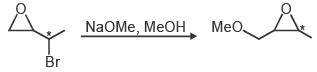*Multiple options can be correct
Chemistry - 2015 Past Year Paper - Question 35

The reaction(s) which give(s) phenol is/are

*Multiple options can be correct
Chemistry - 2015 Past Year Paper - Question 36

Which of the following species is/are aromatic in nature?

*Multiple options can be correct
Chemistry - 2015 Past Year Paper - Question 37

Which of the following compound(s) show(s) only two signals in 1H NMR and a strong IR band at ~1690 cm–1?

*Multiple options can be correct
Chemistry - 2015 Past Year Paper - Question 38

If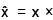and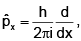then the value(s) of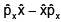is/are

*Multiple options can be correct
Chemistry - 2015 Past Year Paper - Question 39

At what angle(s) of incidence, X- rays of wavelength 5.0 Å will produce diffracted beam from the (110) planes in a simple cubic lattice with a = 10 Å?

*Multiple options can be correct
Chemistry - 2015 Past Year Paper - Question 40

Which of the following thermodynamic relation(s) is/are correct?

*Answer can only contain numeric values
Chemistry - 2015 Past Year Paper - Question 41

Given that the crystal field stabilization energy for [Co(H2O)6]2+ is 7360 cm–1, the calculated value of Δo in kJ mol-1 is _______

*Answer can only contain numeric values
Chemistry - 2015 Past Year Paper - Question 42

The amount (in grams) of potassium dichromate (MW = 294) present in 75 mL of 0.16 M aqueous solution is _______

*Answer can only contain numeric values
Chemistry - 2015 Past Year Paper - Question 43

Given that the expected spin- only magnetic moment for (Et4N)2[NiCl4] is 2.83 μB, the total number of unpaired electrons in this complex is _______

*Answer can only contain numeric values
Chemistry - 2015 Past Year Paper - Question 44

The pKa values of lysine are 2.18, 8.95 and 10.79. The isoelectric point of lysine is _______

*Answer can only contain numeric values
Chemistry - 2015 Past Year Paper - Question 45

The amount (in grams) of NaOH (MW = 40) required for complete neutralization of one mole of the following compound is _______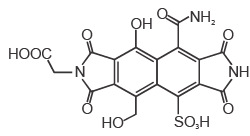*Answer can only contain numeric values
Chemistry - 2015 Past Year Paper - Question 46

In the given list, the total number of compounds that form a clear homogenous solution on treatment with cold dilute H2SO4 is _______

1-propyne
cyclohexanone
cyclohexane
1-propene
propan-1-amine
propoxypropane
tetrahydropyran
ethyl butanoate
pyridine

*Answer can only contain numeric values
Chemistry - 2015 Past Year Paper - Question 47

In the gas phase, the ratio of excluded volume to molecular volume for a spherical molecule is _______

*Answer can only contain numeric values
Chemistry - 2015 Past Year Paper - Question 48

The pKa values of H3PO4 are 2.12, 7.21 and 12.67. The pH of a phosphate buffer containing 0.2 M NaH2PO4 and 0.1 M Na2HPO4 is _______

*Answer can only contain numeric values
Chemistry - 2015 Past Year Paper - Question 49

For the reaction,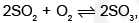the equilibrium constant KP = 5.0 at 207 °C. If the partial pressures of SO2, O2 and SO3 are 1.0 × 10–3, 0.20 and 1.0 × 10–4, respectively, then the Gibbs free energy of the reaction (ΔrG) in kJ mol-1 at 207°C is _________
[Given: R = 8.314 J K-1mol–1]

*Answer can only contain numeric values
Chemistry - 2015 Past Year Paper - Question 50

Two moles of an ideal gas is expanded isothermally and reversibly from 5 to 1 bar at 298 K. The change in the entropy (in J K–1) of the system is _______

*Answer can only contain numeric values
Chemistry - 2015 Past Year Paper - Question 51

The ionic radii of Cs+ and Cl- ions are 181 and 167 pm, respectively. The Born exponents for the He, Ne, Ar, Kr and Xe configurations are 5, 7, 9, 10 and 12, respectively. If the value of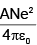is 2.45 × 10–4 J m, the lattice energy (in kJ mol–1) of CsCl according to Born- Landé equation is ________

*Answer can only contain numeric values
Chemistry - 2015 Past Year Paper - Question 52

A 2.5 × 10–4 M solution of a complex exhibits an absorption maximum at 625 nm with an absorbance of 0.90 when measured in a cuvette with a path length of 1.5 cm. The absorbance of 1.5 × 10–3 M solution of the same complex recorded in a cuvette with a path length of 0.2 cm is _______

*Answer can only contain numeric values
Chemistry - 2015 Past Year Paper - Question 53

A wood specimen containing 14C taken from an ancient palace showed 24 counts in 3 minutes per gram of carbon in a detector. However, a fresh wood showed 52 counts in 2 minutes per gram of carbon. Assuming no background signal in the detector and half life of 14C as 5730 years, the age (in years) of the wood specimen is _______

*Answer can only contain numeric values
Chemistry - 2015 Past Year Paper - Question 54

The magnetic field (in Tesla) required for flipping a 1H nucleus in an NMR spectrometer operating at 400 MHz is _______ [Given: g = 2.67 × 108 T–1 s–1, π = 3.14]

*Answer can only contain numeric values
Chemistry - 2015 Past Year Paper - Question 55

The total number of compounds (shown below) that form phenylhydrazone derivatives under acidic conditions is _______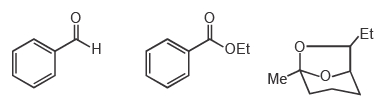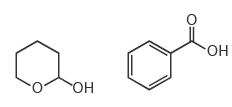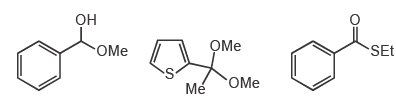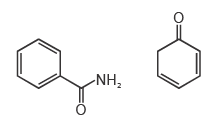*Answer can only contain numeric values
Chemistry - 2015 Past Year Paper - Question 56

The number of possible monoalkylated products formed in the Friedel- Crafts reaction of anisole with 2- chloro- 3- methylbutane in the presence of anhydrous AlCl3 at 50 °C is _______

*Answer can only contain numeric values
Chemistry - 2015 Past Year Paper - Question 57

For a reaction, the rate constant at 25 °C is doubled when the temperature is raised to 45 °C. The activation energy (in kJ mol–1) of the reaction is _______ [Given: ln2 = 0.693]

*Answer can only contain numeric values
Chemistry - 2015 Past Year Paper - Question 58

The standard reduction potentials of the Fe3+/Fe2+ and Fe2+/Fe couples are 0.77 and –0.44 V, respectively. The standard reduction potential (in V) for the Fe3+/Fe couple is _______

*Answer can only contain numeric values
Chemistry - 2015 Past Year Paper - Question 59

When a perfect monolayer of stearic acid is formed at the air- water interface, each molecule of stearic acid (MW = 284, density = 0.94 g cm–3) occupies an area of 20 Å2. The length (in Å) of the molecule is _______

*Answer can only contain numeric values
Chemistry - 2015 Past Year Paper - Question 60

In an ideal monoatomic gas, the speed of sound is given by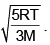If the speed of sound in argon at 25 °C is 1245 km h–1, the root mean square velocity in m s–1 is _______

## IIT JAM Past Year Papers and Model Test Paper (All Branches)

8 docs|48 tests
Information about Chemistry - 2015 Past Year Paper Page
In this test you can find the Exam questions for Chemistry - 2015 Past Year Paper solved & explained in the simplest way possible. Besides giving Questions and answers for Chemistry - 2015 Past Year Paper, EduRev gives you an ample number of Online tests for practice

## IIT JAM Past Year Papers and Model Test Paper (All Branches)

8 docs|48 tests(Scan QR code)